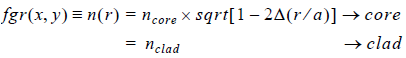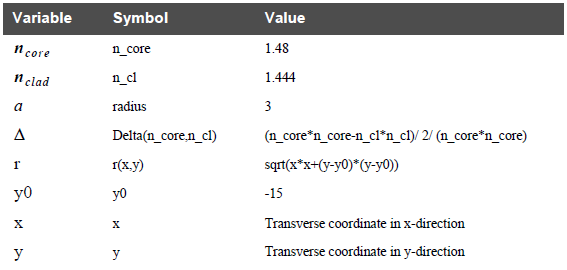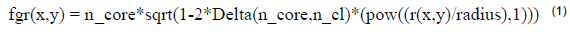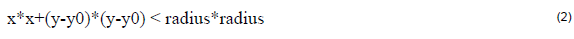The Triangular core fiber can be defined as:To implement the above equation in User Function Profile, we will first explain the User Variables and User Functions (see Table 12).Table 12: User Variables and User Functions

Therefore, Triangular Core Fiber equation can be defined as follows:with the limits as follows: